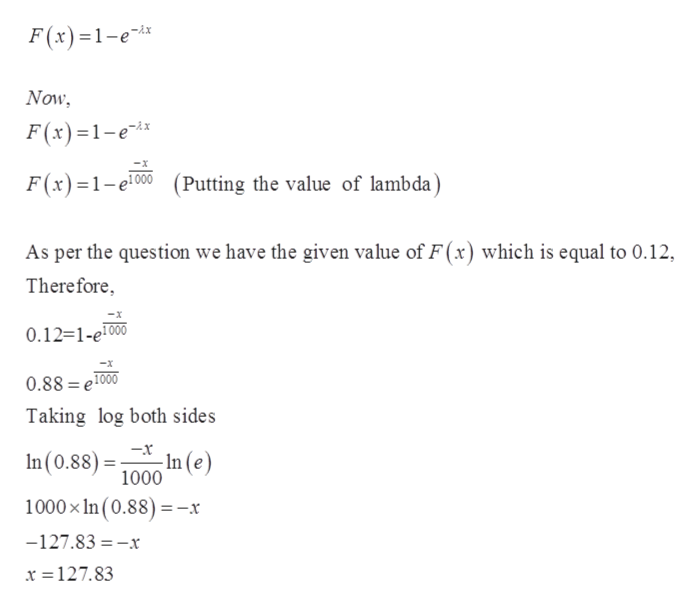The probability density function of the time to failure of an electronic component in a copier (in hours) is f(x) = (e-x/1000 )/1000 for x > 0. Determine the the number of hours at which 12% of all components have failed. Please enter the answer to 2 decimal places.

Question
The probability density function of the time to failure of an electronic component in a copier (in hours) is f(x) = (e-x/1000 )/1000 for x > 0. Determine the the number of hours at which 12% of all components have failed. Please enter the answer to 2 decimal places.

Step 1

The time to failure of an electronic component in copier follows Exponential Distribution with pdf f(x) = (e-x/1000)/1000 for x > 0.

We need to find the value of x ­(number of hours) at which 12% of all component have failed.

Step 2

We have the pdf of exponential distribution but as per the question we need to find the probability that in x hours all components will fail that means we need a cumulative probability till point x.

So, we ...help_outlineImage TranscriptioncloseF(x)1-e -Ax Now F(x)1-e F(x)1e00Putting the value of lambda) As per the question we have the given value of F(x) which is equal to 0.12 Therefore 0.12=1-e1000 0.88 e1000 Taking log both sides In (e) In (0.88)= 1000 -x 1000x In (0.88) -x -127.83 -x x =127.83 fullscreen

Want to see the full answer?

See Solution

Want to see this answer and more?

Our solutions are written by experts, many with advanced degrees, and available 24/7

See Solution
Tagged in

Random Variables